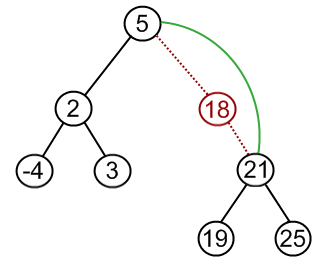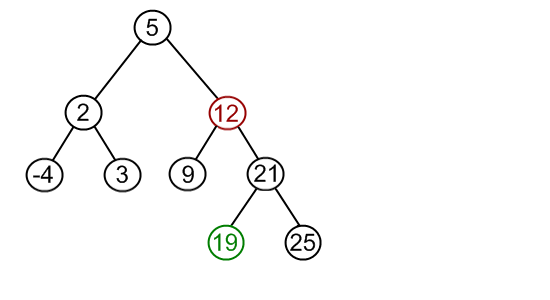# Binary search tree. Removing a node

Remove operation on binary search tree is more complicated, than add and search. Basically, in can be divided into two stages:

• search for a node to remove;
• if the node is found, run remove algorithm.

### Remove algorithm in detail

Now, let's see more detailed description of a remove algorithm. First stage is identical to algorithm for lookup, except we should track the parent of the current node. Second part is more tricky. There are three cases, which are described below.

1. Node to be removed has no children.

This case is quite simple. Algorithm sets corresponding link of the parent to NULL and disposes the node.

Example. Remove -4 from a BST.2. Node to be removed has one child.

It this case, node is cut from the tree and algorithm links single child (with it's subtree) directly to the parent of the removed node.

Example. Remove 18 from a BST.3. Node to be removed has two children.

This is the most complex case. To solve it, let us see one useful BST property first. We are going to use the idea, that the same set of values may be represented as different binary-search trees. For example those BSTs:contains the same values {5, 19, 21, 25}. To transform first tree into second one, we can do following:

• choose minimum element from the right subtree (19 in the example);
• replace 5 by 19;
• hang 5 as a left child.

The same approach can be utilized to remove a node, which has two children:

• find a minimum value in the right subtree;
• replace value of the node to be removed with found minimum. Now, right subtree contains a duplicate!
• apply remove to the right subtree to remove a duplicate.

Notice, that the node with minimum value has no left child and, therefore, it's removal may result in first or second cases only.

Example. Remove 12 from a BST.Find minimum element in the right subtree of the node to be removed. In current example it is 19.Replace 12 with 19. Notice, that only values are replaced, not nodes. Now we have two nodes with the same value.Remove 19 from the left subtree.## Code snippets

First, check first if root exists. If not, tree is empty, and, therefore, value, that should be removed, doesn't exist in the tree. Then, check if root value is the one to be removed. It's a special case and there are several approaches to solve it. We propose the dummy root method, when dummy root node is created and real root hanged to it as a left child. When remove is done, set root link to the link to the left child of the dummy root.

In the languages without automatic garbage collection (i.e., C++) the removed node must be disposed. For this needs, remove method in the BSTNode class should return not the boolean value, but the link to the disposed node and free the memory in BinarySearchTree class.

### Java

public class BinarySearchTree {

public boolean remove(int value) {

if (root == null)

return false;

else {

if (root.getValue() == value) {

BSTNode auxRoot = new BSTNode(0);

auxRoot.setLeftChild(root);

boolean result = root.remove(value, auxRoot);

root = auxRoot.getLeft();

return result;

} else {

return root.remove(value, null);

}

}

}

}

public class BSTNode {

public boolean remove(int value, BSTNode parent) {

if (value < this.value) {

if (left != null)

return left.remove(value, this);

else

return false;

} else if (value > this.value) {

if (right != null)

return right.remove(value, this);

else

return false;

} else {

if (left != null && right != null) {

this.value = right.minValue();

right.remove(this.value, this);

} else if (parent.left == this) {

parent.left = (left != null) ? left : right;

} else if (parent.right == this) {

parent.right = (left != null) ? left : right;

}

return true;

}

}

public int minValue() {

if (left == null)

return value;

else

return left.minValue();

}

}

### C++

bool BinarySearchTree::remove(int value) {

if (root == NULL)

return false;

else {

if (root->getValue() == value) {

BSTNode auxRoot(0);

auxRoot.setLeftChild(root);

BSTNode* removedNode = root->remove(value, &auxRoot);

root = auxRoot.getLeft();

if (removedNode != NULL) {

delete removedNode;

return true;

} else

return false;

} else {

BSTNode* removedNode = root->remove(value, NULL);

if (removedNode != NULL) {

delete removedNode;

return true;

} else

return false;

}

}

}

BSTNode* BSTNode::remove(int value, BSTNode *parent) {

if (value < this->value) {

if (left != NULL)

return left->remove(value, this);

else

return NULL;

} else if (value > this->value) {

if (right != NULL)

return right->remove(value, this);

else

return NULL;

} else {

if (left != NULL && right != NULL) {

this->value = right->minValue();

return right->remove(this->value, this);

} else if (parent->left == this) {

parent->left = (left != NULL) ? left : right;

return this;

} else if (parent->right == this) {

parent->right = (left != NULL) ? left : right;

return this;

}

}

}

int BSTNode::minValue() {

if (left == NULL)

return value;

else

return left->minValue();

}

## Visualizers

1. Binary Search Tree (Delete) in Java Applets Centre

## Recommended books

### Java

public class BinarySearchTree {

public boolean remove(int value) {

if (root == null)

return false;

else {

if (root.getValue() == value) {

BSTNode auxRoot = new BSTNode(0);

auxRoot.setLeftChild(root);

boolean result = root.remove(value, auxRoot);

root = auxRoot.getLeft();

return result;

} else {

return root.remove(value, null);

}

}

}

}

public class BSTNode {

public boolean remove(int value, BSTNode parent) {

if (value < this.value) {

if (left != null)

return left.remove(value, this);

else

return false;

} else if (value > this.value) {

if (right != null)

return right.remove(value, this);

else

return false;

} else {

if (left != null && right != null) {

this.value = right.minValue();

right.remove(this.value, this);

} else if (parent.left == this) {

parent.left = (left != null) ? left : right;

} else if (parent.right == this) {

parent.right = (left != null) ? left : right;

}

return true;

}

}

public int minValue() {

if (left == null)

return value;

else

return left.minValue();

}

}

### C++

bool BinarySearchTree::remove(int value) {

if (root == NULL)

return false;

else {

if (root->getValue() == value) {

BSTNode auxRoot(0);

auxRoot.setLeftChild(root);

BSTNode* removedNode = root->remove(value, &auxRoot);

root = auxRoot.getLeft();

if (removedNode != NULL) {

delete removedNode;

return true;

} else

return false;

} else {

BSTNode* removedNode = root->remove(value, NULL);

if (removedNode != NULL) {

delete removedNode;

return true;

} else

return false;

}

}

}

BSTNode* BSTNode::remove(int value, BSTNode *parent) {

if (value < this->value) {

if (left != NULL)

return left->remove(value, this);

else

return NULL;

} else if (value > this->value) {

if (right != NULL)

return right->remove(value, this);

else

return NULL;

} else {

if (left != NULL && right != NULL) {

this->value = right->minValue();

return right->remove(this->value, this);

} else if (parent->left == this) {

parent->left = (left != NULL) ? left : right;

return this;

} else if (parent->right == this) {

parent->right = (left != NULL) ? left : right;

return this;

}

}

}

int BSTNode::minValue() {

if (left == NULL)

return value;

else

return left->minValue();

}